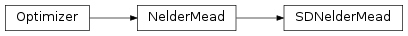# 4.9. `pyopus.optimizer.sdnm` — Unconstrained sufficient descent Nelder-Mead simplex optimizer¶Sufficient descent Nelder-Mead simplex optimizer (Price-Coope-Byatt) (PyOPUS subsystem name: SDNMOPT)

A provably convergent version of the Nelder-Mead simplex algorithm. The algorithm performs unconstrained optimization. Convergence is achieved by imposing sufficient descent on simplex steps and by keeping the simplex internal angles away from 0.

The algorithm was published in

Price C.J., Coope I.D., Byatt D.: A Convergent Variant of the Nelder-Mead Algorithm. Journal of Optimization Theory and Applications, vol. 113, pp. 5-19, 2002.

Byatt D.: Convergent Variants of the Nelder-Mead Algorithm, MSc thesis, University of Canterbury, 2000.

class `pyopus.optimizer.sdnm.``SDNelderMead`(function, debug=0, fstop=None, maxiter=None, reflect=1.0, expand=2.0, outerContract=0.5, innerContract=-0.5, shrink=0.5, reltol=1e-15, ftol=1e-15, xtol=1e-09, simplex=None, kappa=4.0, K0=1000.0, N0=100.0, nu=4.5, tau=1e-18)

Unconstrained sufficient-descent Nelder-Mead optimizer class (Price-Coope-Byatt algorithm)

kappa is the frame shrink factor.

K0 is the maximal length of a vector in basis.

N0 defines initial sufficient descent, which is N0 times smaller than average function difference between the best point and the remaining \$n\$ points of the initial simplex

nu is the exponential (>1) for calculating new sufficient descent.

tau is the bound on basis determinant.

Initial value of h is not given in the paper. The MSc thesis, however, specifies that it is 1.

See the `NelderMead` class for more information.

`check`()

Checks the optimization algorithm’s settings and raises an exception if something is wrong.

`logFactorial`(n)

Calculates log(n!) where log() is the natiral logarithm. Uses Stirling’s approximation for n>50.

`orderSimplex`()

Overrides default sorting in Nelder-Mead simplex.

Reorders the points and the corresponding cost function values of the simplex in such way that the point with the lowest cost function value is the first point in the simplex. Secondary sort key is the number of moves of the point. It increses by 1 every time a point moves and is reset to 0 at simplex reshape. Of two points with same f the one with higher number of moves is first.

`reset`(x0)

Puts the optimizer in its initial state and sets the initial point to be the 1-dimensional array or list x0. The length of the array becomes the dimension of the optimization problem (`ndim` member).

The initial simplex is built around x0 by calling the `buildSimplex()` method with default values for the rel and abs arguments.

If x0 is a 2-dimensional array or list of size (ndim*+1) times *ndim it specifies the initial simplex.

The initial value of the natural logarithm of the simplex side vectors determinant is calculated and stored. This value gets updated at every simplex algorithm step. The only time it needs to be reevaluated is at reshape. But that is also quite simple because the reshaped simplex is orthogonal. The only place where a full determinant needs to be calculated is here.

`reshape`(v)

Reshapes basis given by rows of v into an orthogonal linear base.

Returns a tuple (bnew, logDet) where bnew holds the reshaped basis and logDet is log(n! det([v])).

`run`()

Runs the optimization algorithm.

`sortedSideVectors`()

Returns a tuple (vsorted, lsorted) where vsorted is an array holding the simplex side vectors sorted by their length with longest side first. The first index of the 2-dimensional array is the side vector index while the second one is the component index. lsorted is a 1-dimensional array of corresponding simplex side lengths.

Example file sdnm.py in folder demo/optimizer/

```# Optimize MGH suite with sufficient descent Nelder-Mead optimizer.

from pyopus.optimizer.sdnm import SDNelderMead
from pyopus.optimizer.base import Reporter
from pyopus.problems.mgh import *
from numpy import array, sqrt
from platform import system
if system()=='Windows':
# clock() is the most precise timer in Windows
from time import clock as timer
else:
# time() is the most precise timer in Linux
from time import time as timer
from sys import stdout

# Custom reporter that prints dots
class MyReporter(Reporter):
def __init__(self, name, iterSpacing=1, concise=False, printImprovement=True):
Reporter.__init__(self)
self.name=name
self.iterSpacing=iterSpacing
self.concise=concise
self.printImprovement=printImprovement
self.fbest=None
self.lastPrintout=None

def reset(self):
fbest=None

def __call__(self, x, f, opt):
report=False

if self.fbest is None:
self.lastPrintout=opt.niter
self.fbest=f
report=True

if self.fbest>f and self.printImprovement:
self.fbest=f
report=True

if opt.niter-self.lastPrintout>=self.iterSpacing:
report=True

if report:
if self.concise:
stdout.write(".")
stdout.flush()
else:
print("%30s (%2d) iter=%-6d f=%12.4e fbest=%12.4e" % (self.name[:30], x.size, opt.niter, f, self.fbest))
self.lastPrintout=opt.niter

if __name__=='__main__':
suite=[
[ Rosenbrock() ],
[ FreudensteinAndRoth() ],
[ PowellBadlyScaled() ],
[ BrownBadlyScaled() ],
[ Beale() ],
[ JennrichAndSampson() ],
[ McKinnon() ],
[ McKinnon(), array([[0.0, 0.0], [1.0, 1.0], [(1.0+sqrt(33.0))/8.0, (1.0-sqrt(33.0))/8.0]]) ],
[ HelicalValley() ],
[ Bard() ],
[ Gaussian() ],
[ Meyer() ],
[ GulfResearchAndDevelopement() ],
[ Box3D() ],
[ PowellSingular() ],
[ Wood() ],
[ KowalikAndOsborne() ],
[ BrownAndDennis() ],
[ Quadratic(4) ],
[ PenaltyI(4) ],
[ PenaltyII(4) ],
[ Osborne1() ],
[ BrownAlmostLinear(5) ],
[ BiggsEXP6() ],
[ ExtendedRosenbrock(6) ],
[ BrownAlmostLinear(7) ],
[ Quadratic(8) ],
[ ExtendedRosenbrock(8) ],
[ VariablyDimensioned(8) ],
[ ExtendedPowellSingular(8) ],
[ Watson() ],
[ ExtendedRosenbrock(10) ],
[ PenaltyI(10) ],
[ PenaltyII(10) ],
[ Trigonometric() ],
[ Osborne2() ],
[ ExtendedPowellSingular(12) ],
[ Quadratic(16) ],
[ Quadratic(24) ],
]

results=[]

# Sub-suites of problems
# mysuite=suite[7:8]	# McKinnon (alt)
# mysuite=suite[0:8] # First 8 functions
# mysuite=suite[1:2]	# Freudenstein and Roth
# mysuite=[suite, suite, suite, suite, suite, suite] # brown, mckin, helical, gaussian, kowalik, trig
mysuite=[suite]
mysuite=suite

for probdef in mysuite:
# Take problem function.
prob=probdef

# Write a message.
print("\nProcessing: "+prob.name+" ("+str(prob.n)+") ")

# Create optimizer object.
opt=SDNelderMead(prob.f, debug=0, maxiter=100000)

# Install custom reporter plugin. Print dot for 500 evaluations.
opt.installPlugin(MyReporter(prob.name, 1000, concise=True, printImprovement=False))

# If problem has a custom initial simplex, set it.
if len(probdef)==1:
opt.reset(prob.initial)
else:
# Custom simplex (for McKinnon)
opt.reset(probdef)

# Start timing, run, measure time.
dt=timer()
opt.run()
dt=timer()-dt

# Write number of function evaluations.
print(" %d evaluations" % opt.niter)

# Calculate initial and final gradient
gini=prob.g(prob.initial)
gend=prob.g(opt.x)

# Store results for this problem.
result={
'i': opt.niter,
'x': opt.x,
'f': opt.f,
'gi': sqrt((gini**2).sum()),
'ge': sqrt((gend**2).sum()),
't': dt
}
results.append(result)

# Print summary. Last column is initial/final gradient.
print("\n")
for i in range(0, len(mysuite)):
prob=mysuite[i]
print(
"%2d: %30s (%2d): ni=%6d f=%16.8e gradient: %9.1e -> %9.1e : r=%9.1e" % (
i,
prob.name[:30],
prob.initial.size,
results[i]['i'],
results[i]['f'],
results[i]['gi'],
results[i]['ge'],
results[i]['gi']/results[i]['ge'],
)
)

```